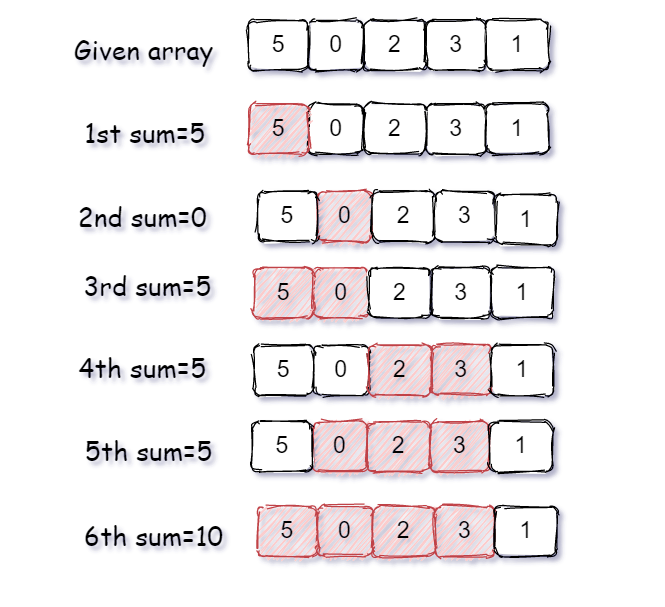38

# Count all sub-arrays having sum divisible by k

Difficulty: MEDIUMContributed By
Ambuj verma
Avg. time to solve
15 min
Success Rate
85%

Problem Statement
Suggest Edit

#### Given an array ‘ARR’ and an integer ‘K’, your task is to find all the count of all sub-arrays whose sum is divisible by the given integer ‘K’.

##### Note:
``````If there exists no subarray in the given sequence whose sum is divisible by ‘K’ then simply return ‘0’.
``````
##### Example:
``````Suppose the given array is ‘ARR’ = { 5, 0, 2, 3, 1} and ‘K = 5’.
As we can see in below image, there are a total of 6 subarrays that have the total sum divisible by ‘K’
So we return the integer 6.
``````##### Input format:
``````The first line of input contains an integer ‘T’ denoting the number of test cases.
The next ‘2*T’ lines represent the ‘T’ test cases.

The first line of each test case contains two space-separated integers the first integer ‘N’ will denote the number of elements in the array and the second integer denotes integer ‘K’.

The second line of each test case contains ‘N’ space-separated integer that is elements of the array.
``````
##### Output Format
``````For each test case, print an integer that is the count of all subarray that sum is divisible by ‘K’.
``````
##### Note:
``````You are not required to print the output explicitly, it has already been taken care of. Just implement the function.
``````
##### Constraints:
``````1 <= T <= 50
1 <= K,N <= 10^4
-10^9 <= ARR[i] <= 10^9

Time limit: 1 second
``````
##### Sample Input 1:
``````2
3 2
2 3 1
4 1
1 2 3 4
``````
##### Sample Output 1:
``````3
10
``````
##### Explanation of sample input 1:
``````Test Case 1:

Given ‘ARR’ is { 2, 3,1 } and ‘K’ is ‘2’.
All the sub-array with sum is divided by ‘K’ are -
{ 2 }  because the sum is 2 and sum 2 is divisible by 2
{ 3, 1 } because the sum is 3 + 1 = 4 and sum 4 is divisible by 2.
{ 2, 3, 1 } because the sum is 2 + 3 + 1 = 6 and sum 6 is divisible by 2.

Hence there is a total of three subarrays that has sum divisible by 2.

Test Case 2:

Given ‘ARR’ is { 1, 2, 3, 4 } and ‘K’ is ‘1’.
Given ‘K’ is 1 that’s why each and every sub-arrays sum will be divisible by ‘1’ and with the size of ‘4’ array total number of subarray possible is ‘( 4*5 /2 ) = 20/2 = 10’.
All possible subarray -
{ 1 }, { 2 }, { 3 }, { 4 }, { 1, 2 }, { 2, 3 }, { 3, 4 }, { 1, 2, 3 }, { 2, 3, 4 }, { 1, 2, 3, 4 } and all subarray sum is divisible by ‘1’.
Hence there are overall 10 subarrays that has sum divisible by ‘1’.
``````
##### Sample Input 2:
``````2
4 3
1 4 5 2
3 2
1 1 2
``````
##### Sample Output 2:
``````2
3
``````Console